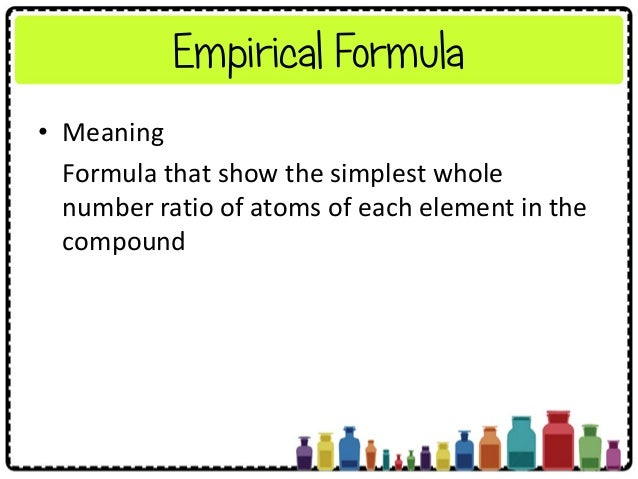Empirical Formulasource : slide share.net

Empirical Formula

“The simplest formula of an organic compound which represents its % composition is called its empirical formula or simple formula”.

1. An organic Compound contains C= 48%, H= 8% . 0.48 gm. of compd was Kjeldahlised & liberated NH3 requires 19.2 ml N/2 H2SO4. Find the empi. formula

Ans.   Normality of acid   =1/2 =0.5

Volume of acid  =19.2 ml

w = mass of compound = 0.48 gm

% of Nitrogen = 1.4 x Normality x Volume /w

=1.4 x 0.5 x 19.2 /0.48

% of Nitrogen= 28 %

% of O= 100– ( sum of % of all other elements present)

% of O= 100–(%of C + % of H + % of nitrogen)

% of O= 100–(48+8+28)

% of O = 16%

 Element % Atomic weight Relative no. of atoms Simplest Ratio C 48 12 48/12=4 4/1=4 H 8 1 8/1=8 8/1=8 N 28 14 28/14=2 2/1=2 O 16 16 16/16=1 1/1=1

C:H:N:O=4:8:2:1

Emp. Formula = C4H8N2O Ans.

Question  –> % of C= 40.68%, H=8.47%, N=23.73% Calculate  Empirical Formula ?

Ans.

% of O= 100– ( sum of % of all other elements present)

% of O= 100–(%of C + % of H + % of nitrogen)

% of O =100–(40.68+8.47+23.73%) =27.12 %

 Element % Atomic weight Relative no. of atoms Simplest Ratio C 40.68 12 40.68/12=3.39 3.39/1.69=2 H 8.47 1 8.47/1=8.47 8.47/1.69=5 N 23.73 14 23.73/14=1.69 1.69/1.169=1 O 27.12 16 27.12/16=1.69 1.69/1.169=1

C:H:N:O=2:5:1:1

Emp. Formula = C2H2NO

Question  —> % of C= 39.13%, H=8.64%, Calculate Empirical Formula?

Solution-

% of O= 100– ( sum of % of all other elements present)

% of O= 100–(%of C + % of H)

% of O =100–(39.13+8.64)

% of O =52.23%

 Element % Atomic weight Relative no. of atoms Simplest Ratio simple whole no.ratio C 39.13 12 39.13/12=3.26 3.26/3.26=1 1×3=3 H 8.64 1 8.64/1=8.64 8.64/3.26=2.65 2.65×3=8 O 52.23 16 52.23/16=3.26 3.26/3.26=1 1×3=3

C:H:O=3:8:3

Emp. Formula = C3H8O3